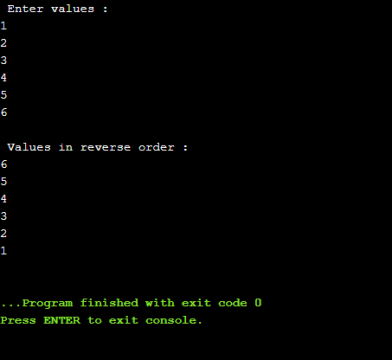C++ Programming

# Pointer Arithmetic in C++ with Programming Examples

## Pointer Arithmetic in C++:

pointer arithmetic in C++:- We can perform two arithmetic operation on pointers. These are addition and subtraction operations. A pointer arithmetic in C++ may be incremented or decremented. It means that we can add or subtract integer value to and from the pointer. Similarly, a pointer arithmetic can be subtracted( or added) from another.

Top Gaming Computers

Best Laptops

Best Graphic Cards

Portable Hard Drives

Best Keyboards

Best High Quality PC Mic

Computer Accessories

The addition and subtraction operation on pointers are different than that of ordinary arithmetic operations. Following set of statements explains the pointer arithmetic in C++:

Int *p;

Int x;

P=&x;

P++;

Suppose the memory address allocated to variable ‘x’ is 200 which is assigned to ‘p’. Both ‘p’ and ‘x’ are of  int type. The size of int type is 2 bytes. When the statement p++ is executed, the contents of p will be 202 (instead of 201). When an integer is added or subtracted from a pointer, the pointer in not incremented or decremented by that integer but is incremented or decremented by integer times the size of the object to which the pointer refers. The size of the object depends upon its data type. So the statement p++ will be evaluated as:

200+1*2=202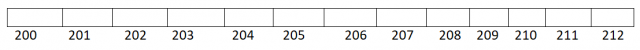Each time a pointer is incremented, it points to the memory location of the next element of its base type. Similarly, each time it is decremented, it points to the location of the previous element. In case of pointers to characters, the increment and decrement operations are performed as normal.

However, all the other pointer variable are incremented or decremented by the size of data type they point to. For example, pointer variable ‘pp’ that points to ‘float’ data type, holds the memory address 4000 and value 2 is added to it as:

pp=pp+2;

after execution the above statement, the value 4008(4000+2*4 = 4008) will be assigned to pp.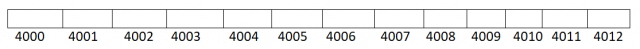A pointer can be assigned to another one if both the pointers are of the same type. Otherwise a cast operator must be used to convert the value of the pointer on the right side of the assignment operator (=) to the pointer type on the left side of the assignment operator.

## Programming Examples:

### Write a program that initializes integer values to an array and displays the value of the array on the screen using pointer notation and pointer arithmetic in C++:

```#include <iostream>
using namespace std;

int main()
{
int a={66,77,44,33,11}, *pa;
pa=a;
cout<<" Value of array "<<endl;
for(int i=0;i<=4;i++)
cout<<" "<<*(pa+i)<<endl;
}
```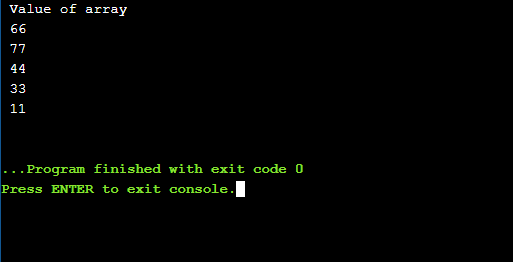### Write a program that inputs values into an array and displays the odd value of array on screen using pointer notation and pointer arithmetic in C++:

```#include <iostream>
using namespace std;

int main()
{
int a, *pa,i;
pa=a;
cout<<"Enter values :"<<endl;
for(i=0;i<=5;i++)
cin>>a[i];
cout<<"\nOdd Values are: "<<endl;
for(i=0;i<=5;i++)
if(*(pa+i)%2==1)
cout<<pa[i]<<endl;
}
```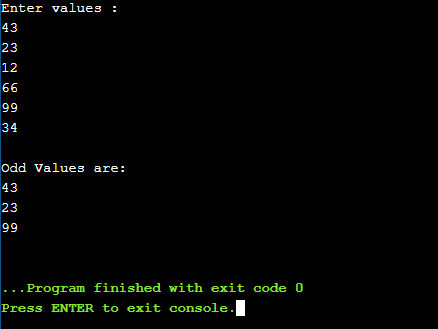### Write a program that inputs value into array and display in reverse order on screen using pointer notation and pointer arithmetic in C++:

```#include <iostream>
using namespace std;

int main()
{
int a, *pa,i;
pa=a;
cout<<" Enter values :"<<endl;
for(i=0;i<=5;i++)
cin>>*pa++;
pa=&a;
cout<<"\n Values in reverse order :"<<endl;
for(i=0; i<=5; i++)
cout<<*pa--<<endl;
}
```